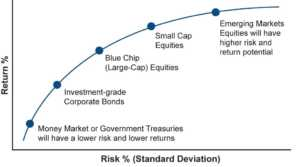The difference between returns on equity and the risk-free rate of return

## What is Equity Risk Premium?

Equity Risk Premium is the difference between returns on equity/individual stock and the risk-free rate of return. The risk-free rate of return, for example, can be benchmarked to longer-term government bonds assuming zero default risk by the government. It is the excess return a stock pays to the holder over and above the risk-free rate for the risk the holder is taking. It is the compensation to the investor for taking a higher level of risk and investing in equity rather than risk-free securities.Equity risk premium and the level of risk are directly correlated. The higher the risk, the higher is the gap between stock returns and the risk-free rate and hence, a higher premium. Empirical data also validates the concept of equity risk premium. It proves that in the longer term, every investor will be rewarded for taking higher risk.

For a rational investor, an increase in risk associated with an investment must be accompanied by a rise in potential reward from that investment for the investment to remain viable. For example, if government bonds are giving an investor 6% returns, any rational investor would choose the stock of any company only if it gave more than 6% returns, say 14%. Here, 14% – 6% = 8% is the equity risk premium.

### Risk-Free Asset

A Risk-Free Asset is an asset whose returns in the future are known with certainty. It comes from complete confidence in the issuer of the asset. We consider government securities to be risk-free assets. The government would, theoretically, never default on the interest and principal payments of it securities. It is because the government has the option of self-financing its debt. It can borrow from the central bank or print more currency. Hence, risk-free assets are exposed to zero default risk and negligible inflation risk.

The formula:

Equity Risk Premium (on the Market) = Rate of Return on the Stock Market − Risk-free Rate

Here, the rate of return on the market can be taken as the return on the concerned index of the relevant stock exchange, i.e., the Dow Jones Industrial Average in the United States. Often, the risk-free rate can be taken as the current rate on long-term government securities.

### Capital Asset Pricing Model and Equity Risk Premium

The Capital Asset Pricing Model, popularly known as CAPM, is a model for calculating the expected returns of a security based on their systematic risk calculated by the Beta coefficient. According to the CAPM, there are two components of returns to an investor. The first component is the time value of money that is given by the risk-free rate of return and the second is the compensation for the risk investors take that is represented by the risk premium.

CAPM can be described using the following equations:

Ra = Rf + βa (RmRf)

Where:

• Ra = Expected return on security ‘a’
• Rf = Risk-free rate of return
• Rm = Expected return on the market
• βa = Beta of ‘a’, and,
• βa = Covariance of Market Return with Stock Return / Variance of Market Return

Here, βa is the risk coefficient of stock ‘a’ which is unique to that particular stock. It measures the stock’s volatility using measures of dispersion.

βa = Covariance of Market Return with Stock Return / Variance of Market Return

Equity Risk Premium = R– Rf = βa (Rm – Rf)

### Numerical Example

Consider the following example. The return on a 10-year government bond is 7%, the beta of security A is 2, and the market return is 12%. Then, the equity risk premium according to the CAPM method is as follows:

βa (Rm – Rf) = 2(12% – 7%) = 10%

• Investing: A Beginner’s Guide
• Bond Pricing